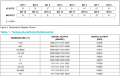# Extracting temperature values from DS18B20

#### AlbertHall

Joined Jun 4, 2014
12,122
I am using a DS18B20 to read a temperature. It is easy to get a float value and then use the normal sprintf to format that into a string. However sprintf uses humungous amounts of flash. I found a much cut down version which doesn't do floats so I want to extract the sign, units, and decimal parts which I can then use with %d in the replacement sprintf. The code below does seem to work but it is horrible. There must be a better way - please!

 This is C code for the microchip XC8 compiler.

Code:
    uint16_t Tem = 0b1111010101011100;
bool Sign = Tem & 0xF000;
char Decimal = ((Tem & 0x000F) * 10) >> 4;
Tem &= 0x0FFF;
Tem = Tem >> 4;
if(Sign)
{
Temp = -Tem;
}
sprintf(LCDbuf, "%3d.%d%cC\0", Temp, Decimal, 0xDF);
The DS18B20 output format is as below:Last edited:

#### upand_at_them

Joined May 15, 2010
923
Your code does work? Negative values are two's compliment, but I don't see you doing the proper conversion.

(By the way, your example temp value is -170.25, which is beyond limit of that sensor.)

#### AlbertHall

Joined Jun 4, 2014
12,122
I got my example reading wrong - the first five bits should all be the same - so my code only works for the wrong example.
But the question remains: how do I extract the parts left and right of the decimal point?

#### upand_at_them

Joined May 15, 2010
923
Code:
Set Sign to "positive".
If the MSb of Temp is 1 then:
Set Sign to "negative".
Invert Temp.
Add 1 to Temp.
You now have the absolute value in Temp and the sign in Sign.
Performing an AND with Temp and 0x000F will give you the decimal portion.
Bitshifting Temp right 4 times will give you the integer portion.

The decimal portion will, of course, have to be multiplied by 0.0625. But a simpler solution might be to use a lookup table on those 16 possible values.

#### 402DF855

Joined Feb 9, 2013
271
Here is one approach that gets rid of sprintf.

C:
const char *dec={
".0",
".0625",
".125",
".1875",
".25",
".3125",
".375",
".4375",
".5",
".5625",
".625",
".6875",
".75",
".8125",
".875",
".9375"
};

char LCDbuf;

void OutputTemp(int16_t val)
{
bool isNeg = (val&0x8000)!=0;
int16_t index=0;
if (isNeg) {
val=-val;
LCDbuf[index++]='-';
}
int16_t frac = val&0xF;
int16_t deg = val>>4;
int16_t ndig=0;
do {
int dig = deg%10;
deg/=10;
LCDbuf[index++] = (char) (dig+'0');
ndig++;
} while (deg);
if (ndig==2) {
char c = LCDbuf[index-1];
LCDbuf[index-1]=LCDbuf[index-2];
LCDbuf[index-2]=c;
} else if (ndig==3) {
char c = LCDbuf[index-1];
LCDbuf[index-1]=LCDbuf[index-3];
LCDbuf[index-3]=c;
}
strcpy(LCDbuf+index,dec[frac]);
}

#### 402DF855

Joined Feb 9, 2013
271
Edit: it is easy to combine the 2/3 digit reversal. Also I'd consider simplifying by showing only the tenths digit which gets rid of strcpy.
C:
const char tenths[]="0112334456678899";
LCDbuf[index++]='.';
LCDbuf[index++]=tenths[frac];
LCDbuf[index]=0;

Last edited:

#### upand_at_them

Joined May 15, 2010
923
Masking the high four bits are unnecessary and generate larger code. Simply test the highest bit, as I proposed above...along with the lookup. Testing the MSb should only generate a "bit test" assembly instruction, instead of the mask approach which is more instructions.

#### AlbertHall

Joined Jun 4, 2014
12,122
With thanks to @402DF855, here is my latest which works for all the values in the datasheet table:
Code:
void OutputTemp(int16_t val)
{
bool isNeg = (val&0x8000)!=0;
int16_t index=0;
if (isNeg) {
val=-val;
LCDbuf[index++]='-';
}
int16_t frac = val&0xF;
int16_t deg = val>>4;
int16_t ndig=0;
do {
int dig = deg%10;
deg/=10;
LCDbuf[index++] = (char) (dig+'0');
ndig++;
} while (deg);

char c = LCDbuf[index-1];
LCDbuf[index-1]=LCDbuf[index-ndig];
LCDbuf[index-ndig]=c;

const char tenths[]="0112334456678899";
LCDbuf[index++]='.';
LCDbuf[index++]=tenths[frac];
LCDbuf[index]=0;
}

#### AlbertHall

Joined Jun 4, 2014
12,122
Incidentally, this means that I also don't need even the cut down sprintf so the result is very small.
Thanks to everyone.

#### 402DF855

Joined Feb 9, 2013
271
Depending on how greedy you are you could check to see if the newest conversion has changed. Obviously this requires LCDbuf not be overwritten between conversions.

C:
void OutputTemp(int16_t val)
{
static int16_t lastval = 0x8000;
if (val==lastval)
return;
lastval=val;
bool isNeg = (val&0x8000)!=0;
...
}